# Coordination geometry

﻿
Coordination geometry

The term coordination geometry is used in a number of related fields of chemistry and solid state chemistry/physics.

## Molecules

The coordination geometry of an atom is the geometrical pattern formed by atoms around the central atom.

## Inorganic coordination complexes

In the field of inorganic coordination complexes it is the geometrical pattern formed by the atoms in the ligands that are bonded to the central atom in a molecule or a coordination complex. The geometrical arrangement will vary according to the number and type of ligands bonded to the metal centre, and to the coordination preference of the central atom, typically a metal in a coordination complex. The number of atoms bonded, (i.e. the number of σ-bonds between central atom and ligands) is termed the coordination number. The geometrical pattern can be described as a polyhedron where the vertices of the polyhedron are the centres of the coordinating atoms in the ligands.

The coordination preference of a metal often varies with its oxidation state. The number of coordination bonds (coordination number) can vary from two as high as 20 in Th(η5-C5H5)4.
One of the most common coordination geometries is octahedral, where six ligands are coordinated to the metal in a symmetrical distribution, leading to the formation of an octahedron if lines were drawn between the ligands. Other common coordination geometries are tetrahedral and square planar.
Crystal field theory may be used to explain the relative stabilities of transition metal compounds of different coordination geometry, as well as the presence or absence of paramagnetism.
VSEPR may be used for complexes of main group element to predict geometry.

## Crystallography usage

In a crystal structure the coordination geometry of an atom is the geometrical pattern of coordinating atoms where the definition of coordinating atoms depends on the bonding model used. For example in the rock salt ionic structure each sodium atom has six near neighbour chloride ions in an octahedral geometry and each chloride has similarly six near neighbour sodium ions in an octahedral geometry. In metals with the body centred cubic (bcc) structure each atom has eight nearest neighbours in a cubic geometry. In metals with the face centred cubic (fcc) structure each atom has twelve nearest neighbours in a cuboctahedral geometry.

## Table of coordination geometries

A table of the coordination geometries encountered is shown below with examples of their occurrence in complexes found as discrete units in compounds and coordination spheres around atoms in crystals (where there is no discrete complex).

Coordination number Geometry Examples of discrete (finite) complex Examples in crystals
2 linear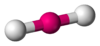Ag(CN)2 in KAg(CN)2  Ag in silver cyanide,
Au in AuI 
3 trigonal planar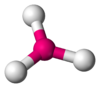Cu(CN)32− in Na2Cu(CN)3.3H2O O in TiO2 rutile structure
4 tetrahedral geometry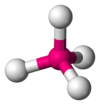CoCl42− Zn and S in zinc sulfide, Si in silicon dioxide
4 square planar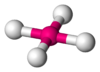AgF4 CuO
5 trigonal bipyramidal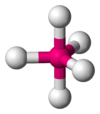SnCl5
5 square pyramidal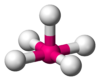InCl52− in (NEt4)2InCl5
6 octahedral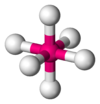Fe(H2O)62+ Na and Cl in NaCl
6 trigonal prismatic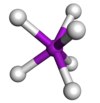Mo(SCHCHS)3 As in NiAs, Mo in MoS2
7 pentagonal bipyramidal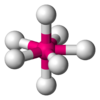ZrF73− in (NH4)3ZrF7 Pa in PaCl5
7 face capped octahedral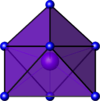[HoIII(PhCOCHCOPh)3(H2O)] La in A-La2O3
7 trigonal prismatic, square face monocapped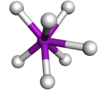TaF72− in K2TaF7
8 cubic Caesium chloride, calcium fluoride
8 square antiprism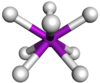TaF83− in Na3TaF8 Thorium(IV) iodide
8 dodecahedral
(note whilst this is term generally
used the correct term is bisdisphenoid
or snub disphenoid as this polyhedron is a deltahedron)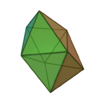Mo(CN)84− in K4[Mo(CN)8].2H2O Zr in K2ZrF6
8 hexagonal bipyramidalN in Li3N
8 octahedral, trans-bicapped Ni in nickel arsenide, NiAs; 6 As neighbours + 2 Ni capping
8 trigonal prismatic, triangular face bicapped Ca in CaFe2O4
8 trigonal prismatic, square face bicapped PuBr3
9 trigonal prismatic, square face tricapped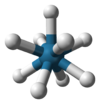[ReH9]2− in potassium nonahydridorhenate SrCl2.6H2O, Th in RbTh3F13
9 monocapped square antiprismatic[Th(tropolonate)4(H2O)] La in LaTe2
10 bicapped square antiprismatic Th(C2O4)42− 
11 Th in [ThIV(NO3)4(H2O)3] (NO3 is bidentate) 
12 icosahedron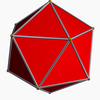Th in Th(NO3)62− ion in Mg[Th(NO3)6].8H2O 
12 cuboctahedron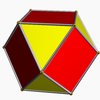ZrIV3−(BH4)4) atoms in fcc metals e.g. Ca
12 anticuboctahedron, triangular orthobicupola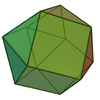atoms in hcp metals e.g. Sc 
14 bicapped hexagonal antiprismatic U(BH4)4

## Naming of inorganic compounds

IUPAC have introduced the polyhedral symbol as part of their IUPAC nomenclature of inorganic chemistry 2005 recommendations to describe the geometry around an atom in a compound.
IUCr have proposed a symbol which is shown as a superscript in square brackets in the chemical formula. For example CaF2 would be Ca[8cb]F2[4t], where [8cb] means cubic coordination and [4t] means tetrahedral. The equivalent symbols in IUPAC are CU−8 and T−4 respectively.
The IUPAC symbol is applicable to complexes and molecules whereas the IUCr proposal applies to crystalline solids.

Wikimedia Foundation. 2010.

### Look at other dictionaries:

• Coordination complex — Cisplatin, PtCl2(NH3)2 A platinum atom with four ligands In chemistry, a coordination complex or metal complex, is an atom or ion (usually metallic), bonded to a surrounding array of molecules or anions, that are in turn known as ligands or… …   Wikipedia

• Coordination polymer — A coordination polymer is an inorganic or organometallic polymer structure containing metal cation centers linked by ligands, extending in an array. It can also be described as a polymer whose repeat units are coordination complexes. Similar… …   Wikipedia

• coordination compound — Chem. complex (def. 10). Also called coordination complex. * * * ▪ chemistry Introduction  any of a class of substances with chemical structures in which a central metal atom is surrounded by nonmetal atoms or groups of atoms, called ligands… …   Universalium

• Coordination sphere — cis [CoCl2(NH3)4]+ The colored atoms form a coordination sphere around the ion of cobalt In coordination chemistry, the coordination sphere refers to a central atom or ion and an array of molecules or anions, the ligands, around. [ …   Wikipedia

• Coordination polymers — Coordination polymer is the term given in inorganic chemistry to a metal coordination compound where a ligand bridges between metal centres, where each metal centre binds to more than one ligand to create an infinite array of metal centres e.g. a …   Wikipedia

• Coordination numbers and geometries of metal cyanide complexes — ▪ Table Coordination numbers and geometries of metal cyanide complexes electron configuration* metal ion cyanide complex geometry total number of valence electrons d2 Mo4+ [Mo(CN)8]4− dodecahedral 18 d3 Cr3+ [Cr(CN)6]3− octahedral 15 d4 Mn3+… …   Universalium

• Octahedral molecular geometry — Idealized structure of a compound with octahedral coordination geometry. In chemistry, octahedral molecular geometry describes the shape of compounds where in six atoms or groups of atoms or ligands are symmetrically arranged around a central… …   Wikipedia

• Polyèdre de coordination — La notion de polyèdre de coordination est utilisée en cristallographie et en chimie pour décrire l environnement d une espèce chimique par ses ligands dans un cristal ou un complexe. Il s agit du polyèdre formé par les ligands autour de l espèce… …   Wikipédia en Français

• Square planar molecular geometry — The square planar molecular geometry in chemistry describes the stereochemistry (spatial arrangement of atoms) that is adopted by certain chemical compounds. As the name suggests, molecules of this geometry have their atoms positioned at the… …   Wikipedia

• Constrained geometry complex — The term constrained geometry complex (CGC) (sometimes also referred as Bercaw s Complex) was first used in a patent issued by the Dow Chemical Co. for complexes in which a pi bonded moiety (e.g. cyclopentadienyl) is linked to one of the other… …   Wikipedia